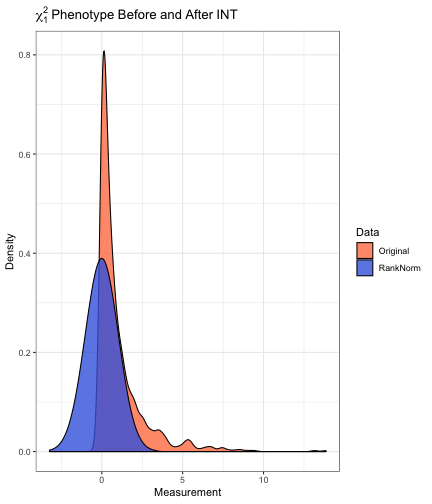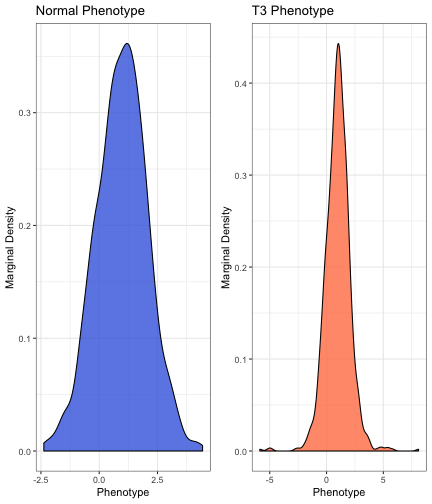# Setting

Consider genetic association analysis with a continuous trait. If the residual distribution is asymmetric (skewed) or diffuse (kurtotic) relative to the normal distribution, then standard linear regression may fail to control the type I error in moderate samples. Even if the sample is sufficiently large for standard linear regression to provide valid inference, it is not fully efficient when the residual distribution is non-normal. Examples of traits that may exhibit non-normal residual distributions include body mass index, circulating metabolites, gene expression, polysomnography signals, and spirometry measurements. In such cases, the rank based inverse normal transformation (INT) has been used to counteract departures from normality. During INT, the sample measurements are first mapped to the probability scale, by replacing the observed values with fractional ranks, then transformed into Z-scores using the probit function. In the following example, a sample of size $$n=1000$$ is drawn from the $$\chi_{1}^{2}$$ distribution. After transformation, the empirical distribution of the measurements in is indistinguishable from standard normal.

library(RNOmni);
# Sample from the chi-1 square distribution
y = rchisq(n=1000,df=1);
# Rank-normalize
z = rankNorm(y);# Data

## Simulated Data

Here, data are simulated for $$n=10^{3}$$ subjects. Genotypes are drawn for $$10^{3}$$ loci in linkage equilibrium with minor allele frequency between 0.05 and 0.50. The model matrix X contains an intercept, four standard normal covariates Z, and the first four genetic principal components. The intercept is set to one, and the remaining regression coefficients are simulated as random effects. The proportion of phenotypic variation explained by covariates is 20%, while the proportion of variation explained by principal components is 5%. Two phenotypes with additive residuals are simulated. The first yn has standard normal residuals, while the second yt has $$t_{3}$$ residuals, scaled to have unit variance.

set.seed(100);
# Sample size
n = 1e3;
## Simulate genotypes
maf = runif(n=1e3,min=0.05,max=0.50);
G = sapply(maf,function(x){rbinom(n=n,size=2,prob=x)});
storage.mode(G) = "numeric";
# Genetic principal components
S = svd(scale(G))\$u[,1:4];
S = scale(S);
# Covariates
Z = scale(matrix(rnorm(n*4),nrow=n));
# Overall design
X = cbind(1,Z,S);
# Coefficient
b = c(1,rnorm(n=4,sd=1/sqrt(15)),rnorm(n=4,sd=1/sqrt(60)));
# Linear predictor
h = as.numeric(X%*%b);
# Normal phenotype
yn = h+rnorm(n);
# T-3 phenotype
yt = h+rt(n,df=3)/sqrt(3);## Data Formatting

The outcome y is expected as a numeric vector. Genotypes G are expected as a numeric matrix, with subjects are rows. If adjusting for covariates or population structure, X is expected as a numeric matrix, which should contain an intercept. Factors and interactions should be expanded in advance, e.g. using model.matrix. Missingness is not expected in either the outcome vector y or the model matrix X, however the genotype matrix G may contain missingness. Observations with missing genotypes are excluded from association testing only at those loci where the genotype is missing.

# Basic Association Test

The basic association test is linear regression of the (untransformed) phenotype on genotype and covariates. BAT provides an efficient implementation using phenotype y, genotypes G, and model matrix X. Standard output includes the score statistic, its standard error, the Z-score, and a $$p$$-value, with one row per column of G. Setting test="Wald" specifies a Wald test. The Wald test may provide more power, but is generally slower. Setting simple=T returns the $$p$$-values only.

# Basic Association Test, Normal Phenotype
Results1 = BAT(y=yn,G=G,X=X);
cat("BAT Applied to Normal Phenotype\n");
cat("\n");
# Basic Association Test, T3 Phenotype
cat("BAT Applied to T3 Phenotype\n");
Results2  = BAT(y=yt,G=G,X=X);

## BAT Applied to Normal Phenotype
##     Score     SE      Z     p
## 1  16.048 18.287  0.878 0.380
## 2  -7.610 16.765 -0.454 0.650
## 3  16.858 21.228  0.794 0.427
## 4 -14.074 11.949 -1.178 0.239
## 5  37.958 19.903  1.907 0.057
## 6  -9.896 20.546 -0.482 0.630
##
## BAT Applied to T3 Phenotype
##     Score     SE      Z     p
## 1   9.510 18.754  0.507 0.612
## 2 -33.482 17.193 -1.947 0.052
## 3  16.044 21.770  0.737 0.461
## 4  -5.921 12.254 -0.483 0.629
## 5  35.560 20.411  1.742 0.082
## 6  -7.839 21.070 -0.372 0.710


# Inverse Normal Transformation

Suppose that a continuous measurement $$u_{i}$$ is observed for each of $$n$$ subjects. Let $$\text{rank}(u_{i})$$ denote the sample rank of $$u_{i}$$ when the measurements are placed in ascending order. The rank based inverse normal transformation (INT) is defined as:

$\text{INT}(u_{i}) = \Phi^{-1}\left[\frac{\text{rank}(u_{i})-k}{n-2k+1}\right]$

Here $$\Phi^{-1}$$ is the probit function, and $$k\in(0,½)$$ is an adjustable offset. By default, the Blom offset of $$k=3/8$$ is adopted.

# Direct INT

In direct INT (D-INT), the INT-transformed phenotype is regressed on genotype and covariates. D-INT is most powerful when the phenotype could have arisen from a rank-preserving transformation of a latent normal trait. DINT implements the association test using phenotype y, genotypes G, and model matrix X. Standard output includes the test statistic, its standard error, the Z-score, and a $$p$$-value, with one row per column of G. Wald and score tests are available. Setting simple=T returns the $$p$$-values only.

# Direct INT Test, Normal Phenotype
cat("D-INT Applied to Normal Phenotype\n");
Results1 = DINT(y=yn,G=G,X=X);
cat("\n");
# Direct INT Test, T3 Phenotype
cat("D-INT Applied to T3 Phenotype\n");
Results2 = DINT(y=yt,G=G,X=X);

## D-INT Applied to Normal Phenotype
##     Score     SE      Z     p
## 1  19.553 20.000  0.978 0.328
## 2  -7.857 18.335 -0.429 0.668
## 3  17.727 23.216  0.764 0.445
## 4 -13.758 13.068 -1.053 0.293
## 5  42.639 21.767  1.959 0.050
## 6 -11.649 22.470 -0.518 0.604
##
## D-INT Applied to T3 Phenotype
##     Score     SE      Z     p
## 1   8.797 21.012  0.419 0.676
## 2 -35.226 19.263 -1.829 0.068
## 3   5.830 24.391  0.239 0.811
## 4  -8.857 13.729 -0.645 0.519
## 5  41.286 22.868  1.805 0.071
## 6  -8.107 23.607 -0.343 0.731


# Indirect INT

In indirect INT (I-INT), the phenotype and genotypes are first regressed on covariates to obtain residuals. The phenotypic residuals are rank normalized. Next, the INT-transformed phenotypic residuals are regressed on genotypic residuals. I-INT is most powerful when the phenotype is linear in covariates, but the residual distribution is skewed or kurtotic. IINT implements the association test, using phenotype y, genotypes G, and model matrix X. Standard output includes the test statistic, its standard error, the Z-score, and a $$p$$-value, with one row per column of G. Setting simple=T returns the $$p$$-values only.

# Indirect INT Test, Normal Phenotype
cat("I-INT Applied to Normal Phenotype\n");
Results1 = IINT(y=yn,G=G,X=X);
cat("\n");
# Indirect INT Test, T3 Phenotype
cat("I-INT Applied to T3 Phenotype\n");
Results2 = IINT(y=yt,G=G,X=X);

## I-INT Applied to Normal Phenotype
##     Score     SE      Z     p
## 1  15.742 17.808  0.884 0.377
## 2  -7.849 16.326 -0.481 0.631
## 3  16.893 20.672  0.817 0.414
## 4 -13.920 11.636 -1.196 0.232
## 5  36.009 19.382  1.858 0.063
## 6 -10.685 20.008 -0.534 0.593
##
## I-INT Applied to T3 Phenotype
##     Score     SE      Z     p
## 1   6.375 17.808  0.358 0.720
## 2 -28.288 16.326 -1.733 0.083
## 3  10.525 20.672  0.509 0.611
## 4  -5.143 11.636 -0.442 0.658
## 5  33.453 19.382  1.726 0.084
## 6  -7.526 20.008 -0.376 0.707


# Omnibus INT

Since neither D-INT nor I-INT is uniformly most powerful, the INT omnibus test (O-INT) adaptively combines them into a single robust and statistically powerful test. Internally, OINT applies both D-INT and I-INT. The corresponding p-values are transformed into Cauchy random deviates, which are averaged to form the omnibus statistic. In general the omnibus p-value will be between the D-INT and I-INT p-values, but closer to smaller of these two. OINT implements the omnibus test, using phenotype y, genotypes G, and model matrix X. The standard output includes the $$p$$-values estimated by each of D-INT, I-INT, and O-INT. Setting simple=T returns the O-INT $$p$$-values only.

# Omnibus INT Test, Normal Phenotype
cat("O-INT Applied to Normal Phenotype\n");
Results1 = OINT(y=yn,G=G,X=X);
cat("\n");
# Omnibus INT Test, T3 Phenotype
cat("O-INT Applied to T3 Phenotype\n");
Results2 = OINT(y=yt,G=G,X=X);

## O-INT Applied to Normal Phenotype
##   DINT-p IINT-p OINT-p
## 1  0.328  0.377  0.352
## 2  0.668  0.631  0.650
## 3  0.445  0.414  0.429
## 4  0.293  0.232  0.259
## 5  0.050  0.063  0.056
## 6  0.604  0.593  0.599
##
## O-INT Applied to T3 Phenotype
##   DINT-p IINT-p OINT-p
## 1  0.676  0.720  0.699
## 2  0.068  0.083  0.075
## 3  0.811  0.611  0.737
## 4  0.519  0.658  0.593
## 5  0.071  0.084  0.077
## 6  0.731  0.707  0.719


# Notes

## Parallelization

All association tests have the option of being run in parallel. To do so, register a parallel backend, e.g. doMC::registerDoMC(cores=4), then specify the parallel=T option.